# NCERT Solutions for Class 10 Maths Exercise 3.1## myCBSEguide App

CBSE, NCERT, JEE Main, NEET-UG, NDA, Exam Papers, Question Bank, NCERT Solutions, Exemplars, Revision Notes, Free Videos, MCQ Tests & more.

NCERT solutions for Maths Pair of Linear Equations in Two Variables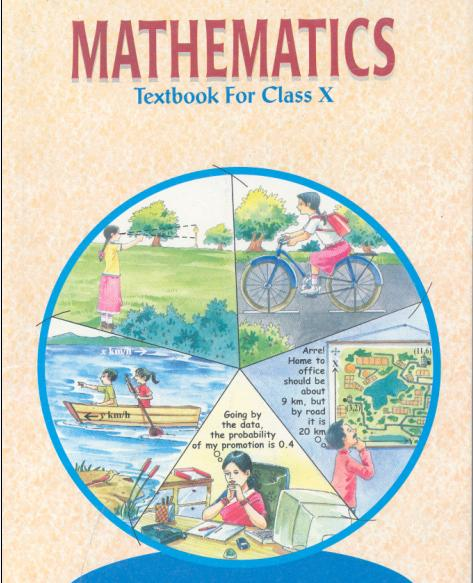## NCERT Solutions for Class 10 Maths Pair of Linear Equations in Two Variables

###### 1. Aftab tells his daughter, “Seven years ago, I was seven times as old as you were then. Also, three years from now, I shall be three times as old as you will be.” (Isn’t this interesting?) Represent this situation algebraically and graphically.

Ans. Let the present age of Aftab and his daughter be x and y respectively.
Seven years ago, Age of Aftab = x – 7 and Age of his daughter = y – 7
According to the given condition,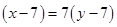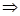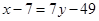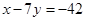Again, Three years hence, Age of Aftab = x + 3 and Age of his daughter = y + 3
According to the given condition,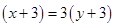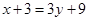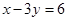Thus, the given conditions can be algebraically represented as:
x – 7y = –42x = –42 + 7y
Three solutions of this equation can be written in a table as follows: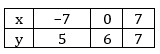And x – 3y = 6x = 6 + 3y
Three solutions of this equation can be written in a table as follows: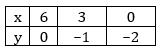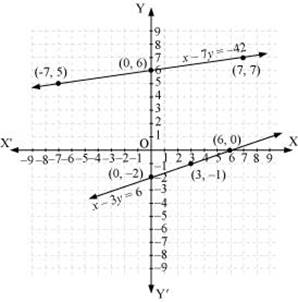The graphical representation is as follows:

Concept insight: In order to represent the algebraic equations graphically the solution set of equations must be taken as whole numbers only for the accuracy. Graph of the two linear equations will be represented by a straight line.

NCERT Solutions for Class 10 Maths Exercise 3.1

###### 2. The coach of a cricket team buys 3 bats and 6 balls for Rs 3900. Later, she buys another bat and 3 more balls of the same kind for Rs 1300. Represent this situation algebraically and graphically.

Ans. Let cost of 1 cricket bat = Rs x and let cost of 1 cricket ball= Rs y

According to given conditions, we have

3x + 6y = 3900 ⇒ x + 2y = 1300… (1)

And x + 3y = 1300… (2)

To represent them graphically, we will find 3 sets of points which lie on the lines.

For equation x + 2y = 1300, we have following points which lie on the line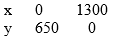For equation x + 3y = 1300, we have following points which lie on the line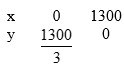We plot the points for both of the equations and it is the graphical representation of the given situation.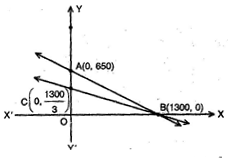It is clear that these lines intersect at B (1300,0).

NCERT Solutions for Class 10 Maths Exercise 3.1

###### 3. The cost of 2 kg of apples and 1 kg of grapes on a day was found to be Rs 160. After a month, the cost of 4 kg of apples and 2 kg of grapes is Rs 300. Represent the situation algebraically and geometrically.

Ans. Let cost of 1 kg of apples = Rs x and let cost of 1 kg of grapes= Rs y

According to given conditions, we have

2x + y = 160… (1)

4x + 2y = 300

⇒ 2x + y = 150… (2)

So, we have equations (1) and (2), 2x + y = 160 and 2x + y = 150 which represent given situation algebraically.

For equation 2x + y = 160, we have following points which lie on the line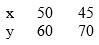For equation 2x + y = 150, we have following points which lie on the line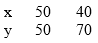We plot the points for both of the equations and it is the graphical representation of the given situation.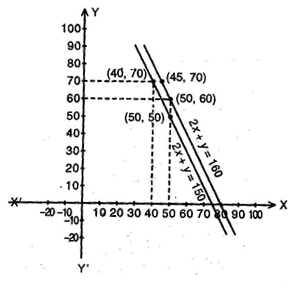## NCERT Solutions for Class 10 Maths Exercise 3.1

NCERT Solutions Class 10 Maths PDF (Download) Free from myCBSEguide app and myCBSEguide website. Ncert solution class 10 Maths includes text book solutions from Mathematics Book. NCERT Solutions for CBSE Class 10 Maths have total 15 chapters. 10 Maths NCERT Solutions in PDF for free Download on our website. Ncert Maths class 10 solutions PDF and Maths ncert class 10 PDF solutions with latest modifications and as per the latest CBSE syllabus are only available in myCBSEguide.

## CBSE app for Class 10

To download NCERT Solutions for Class 10 Maths, Computer Science, Home Science,Hindi ,English, Social Science do check myCBSEguide app or website. myCBSEguide provides sample papers with solution, test papers for chapter-wise practice, NCERT solutions, NCERT Exemplar solutions, quick revision notes for ready reference, CBSE guess papers and CBSE important question papers. Sample Paper all are made available through the best app for CBSE students and myCBSEguide website.### 9 thoughts on “NCERT Solutions for Class 10 Maths Exercise 3.1”

1. cbse guide app is help me thank you

2. Nice

3. thank you

4. Thanks for this Solutions!!!

5. Thanks for this Solutions!!!

6. Thank you for my help

7. This blog’s help me continuously

8. Thank you to help me?????

9. Thanks it is helpful for me